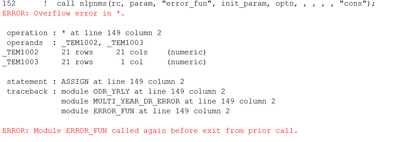## Overflow in matrix multiplication

Hi,

I have some code that tries to minimize a function.

The code is below:

``````start odr_yrly (matrix, year);
yr_odr = j(nrow(matrix), year + 1, 0);
yr_odr[,1] = matrix[,ncol(matrix)];
do i = 1 to year;
yr_odr[,i + 1] = matrix ** 4 * yr_odr[,i];
end;
yr_odr = yr_odr[,2:6];
return(yr_odr);
finish;

start multi_year_dr_error(odr, full_matrix, year, pop);
odr_last = j(1,ncol(odr),1);
odr_full = odr // odr_last;
pop_dist = pop[,5] // {0};
odr_eff = odr_full[,3:6];
idx = loc(odr_eff > 0);
log_odr	= j(nrow(odr_eff),ncol(odr_eff),0);
if ncol(idx) > 0 then log_odr[idx] = log(odr_eff[idx]);
odr_est = odr_yrly(full_matrix, year);
idx2 = loc(odr_est > 0);
log_odr_est	= j(nrow(odr_est),ncol(odr_est),0);
if ncol(idx2) > 0 then log_odr_est[idx2] = log(odr_est[idx2]);
diff = (log_odr - log_odr_est[,2:5]) # pop_dist;
print log_odr diff pop_dist log_odr_est;
diff_sq = diff ## 2;
ssq = diff_sq[+];
return(ssq);
finish;``````

Now I can't see any problem with my matrix multiplication line `yr_odr[,i + 1] = matrix ** 4 * yr_odr[,i];`

however it is giving me errors saying it overflowed.anyone has any idea why that is the case? Happy to post the full optimisation code here but its some long code with multiple parts to the optimisation.

Regards,

Cherrie

1 ACCEPTED SOLUTION

Accepted Solutions

## Re: Overflow in matrix multiplication

The error occurs while you are attempting a nonlinear optimization with a nonlinear constraint. Without seeing the full program, I cannot determine why the error occurs.

4 REPLIES 4

## Re: Overflow in matrix multiplication

The syntax "matrix ** 4" is taking the 4th power of the matrix - so the whole matrix multiplied by itself 4 times.  This might not be what you intend and could potentially lead to an overflow.

## Re: Overflow in matrix multiplication

Hi,

Thanks for your reply. But this is what I intend to do though. However wouldn't overflow be on numbers that are extremely large? My matrix is a transition probability matrix with all entries less than 1...

That's why I don't understand the error and how to solve it.

## Re: Overflow in matrix multiplication

The error occurs while you are attempting a nonlinear optimization with a nonlinear constraint. Without seeing the full program, I cannot determine why the error occurs.

## Re: Overflow in matrix multiplication

Thanks for the reply. I solved this issue.

What happens is I need to trial and error the migration matrix which supposed to be sum to 1 and have values between 0 and 1. however the optimisation program did not have this constraint programmed in so it gives me really large matrices (with individual cell even more than 3e60) which I used to 4th power. This resulted in an overflow.

I have customised the error function so that when row sums of the matrix are more than 1 I force the error function to a really large value. This resolved this issue. Thanks for the reply!

From The DO Loop